# Program to check Duck Number In Java

In this tutorial you will learn about the Program to check Duck Number In Java and its application with practical example.

In this tutorial, we will learn to create a Java Program to check Duck Numbers in Java using Java programming.

## Prerequisites

Before starting with this tutorial we assume that you are best aware of the following Java programming topics:

• Java Operators.
• Basic Input and Output function in Java.
• Class and Object in Java.
• Basic Java programming.
• If-else statements in Java.
• For loop in Java.

## What is a Duck Number?

Duck number is a special positive number that contains zero in within the number except starting are called a Duck number.

Example:

1202

## Program to check Duck Number In Java

In this  program we will find given number is a Evil number or not in using java programming.We would first declared and initialized the required variables. Next, we would prompt user to input the a number.Later we  find number is Evil number or not let’s have a look at the code.

## Output

Duck numberNot a Duck Number.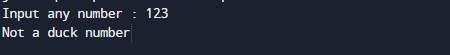In the above program, we have first declared and initialized a set variables required in the program.

• numToString  = it will hold entered number.
• l= it will hold length of a string.
• ch= it will charters of a string.
• c= it will count zeros in numbers.

After declaring variables in the next statement user will be prompted to enter a value and  which will be assigned to variable ‘number’.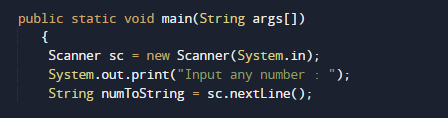Here we will convert given number into string as shown in picture above after that we declare c,ch and l variables to hold value temporary l for length , c for number of zeros in number and ch for holding character of a string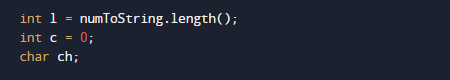after that with the help of while loop we search number of zeros in a string if zeros are present in string increase the counter  value of c to one(c++).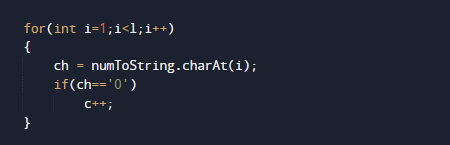and finally we will check if if statement that the value of c is grater than zero and zero is not present at first location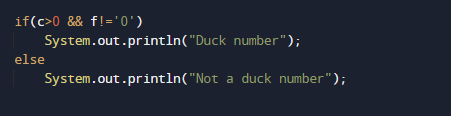if both condition satisfied then the number is Duck number and if not then it not a duck number.

In this tutorial we have learn about the Program to check Duck Number In Java and its application with practical example. I hope you will like this tutorial.